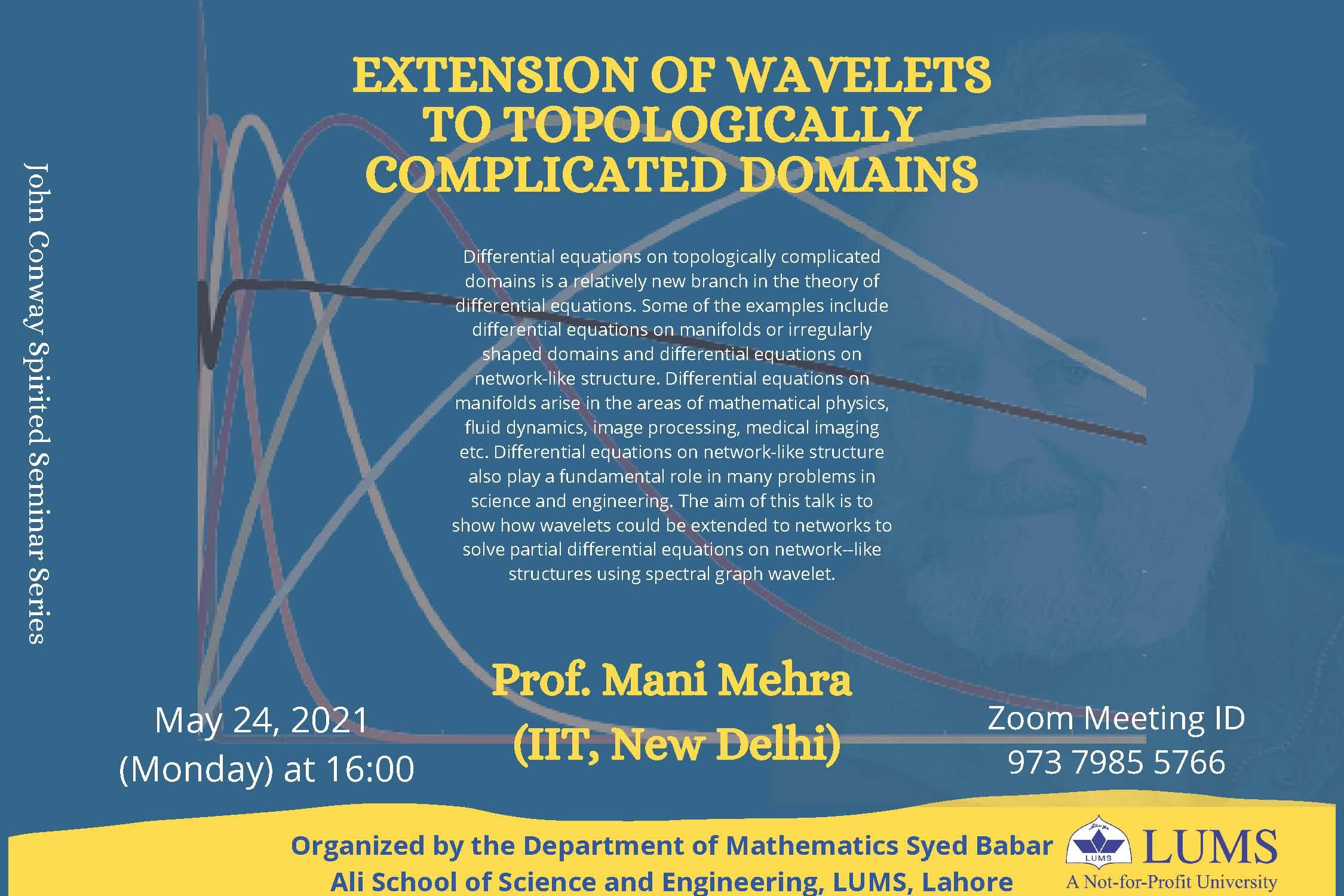Event date:
May 24 2021 4:00 pm

## Extension of Wavelets to Topologically Complicated Domains

Speaker(s)
Prof. Mani Mehra
Venue
Zoom/Online
Abstract
“Differential equations on topologically complicated domains” is a relatively new branch in the theory of differential equations. Some of the examples include differential equations on manifolds or irregularly shaped domains and differential equations on network-like structure. Differential equations on manifolds arise in the areas of mathematical physics, fluid dynamics, image processing, medical imaging etc. Differential equations on network-like structure also play a fundamental role in many problems in science and engineering. The aim of this talk is to show how wavelets could be extended to networks to solve partial differential equations on network – like structures using spectral graph wavelet.

Prof. Mani Mehra (IIT, New Delhi) is going to be our distinguished guest speaker in John Conway Spirited Mathematics Seminar Series. Prof.  Mani Mehra has her research interests in wavelet methods for PDEs and numerical methods.

She will talk on ‘Extension of wavelets to topologically complicated domains’ where she discusses how wavelets could be extended to networks to solve partial differential equations on network--like structures using spectral graph wavelet.